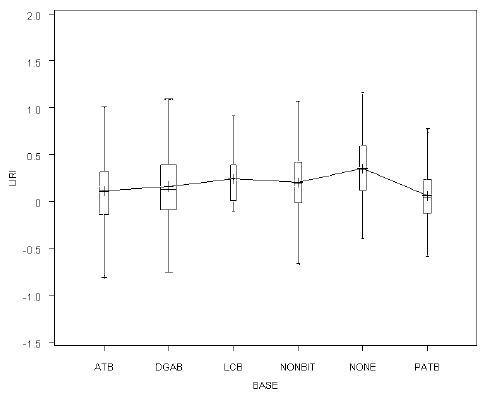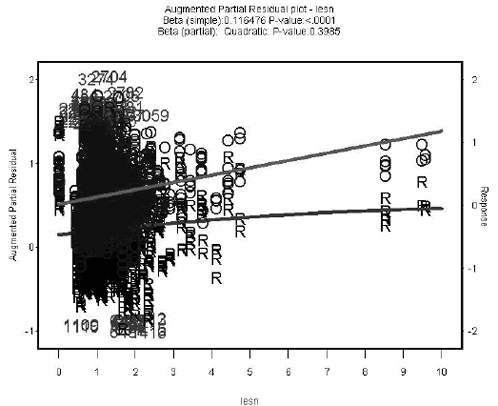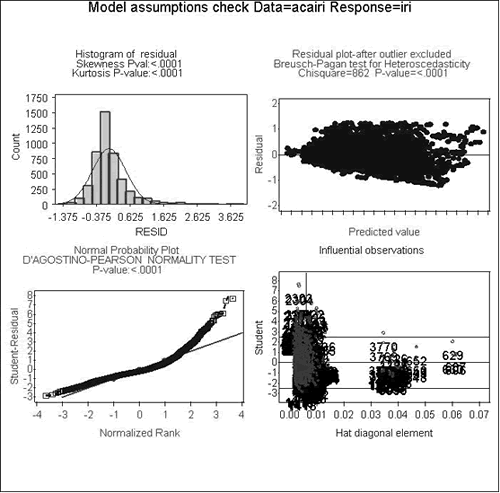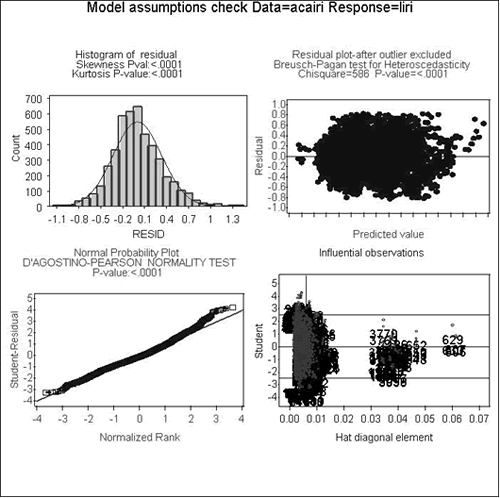U.S. Department of Transportation
1200 New Jersey Avenue, SE
Washington, DC 20590
202-366-4000

Federal Highway Administration Research and Technology
Coordinating, Developing, and Delivering Highway Transportation InnovationsThis report is an archived publication and may contain dated technical, contact, and link information
 E: Inetpub Wwwroot Fhwaroot Publications > Research Publications > LTPP Publications > 06121 > Long-Term Pavement Performance (LTPP) Data Analysis Support: National Pooled Fund Study Tpf-5(013)
 Publication Number: FHWA-HRT-06-121 Date: November 2006

# Long-Term Pavement Performance (LTPP) Data Analysis Support: National Pooled Fund Study Tpf-5(013)

## Chapter 4. Model Fitting Statistical Approach

This section describes the overall procedure for developing regression models for each of the performance measures considered in the study. The intent of the process was to generate a model with the best prediction capability while ensuring assumptions inherent in the process were not violated. Statistical analysis was performed using SAS® software, version 9.1.3.(14)

All explanatory variables discussed in previous sections of this report were included in the initial regression analysis. These variables were both continuous (i.e., FI) and categorical (i.e., BASE) factors. Table 14 provides a summary of all variables considered in the study as well as details on the format of each parameter.

Table 14. Summary of explanatory variables.
Explanatory VariableParameter Type
Pavement Structure Categorical
Freezing Index (FI) Continuous
Freeze-Thaw Cycles (FTC) Continuous
Cooling Index (CI) Continuous
Annual Precipitation (PRECIP) Continuous
Pavement Age (AGE) Continuous
Subgrade Type (SG) Categorical
Base Type (BASE) Categorical
Asphalt Cement Concrete Thickness (ACTHICK) Continuous
Slab Thickness (D) Continuous
Traffic Loading/Structural Capacity Ration (LESN or LEDT) Continuous

An initial investigation was performed on each of the predictor variables to gain an understanding of the range present in the dataset and the nature of the parameters to be used in the regression modeling. Graphical techniques and descriptive statistical measures were used for this evaluation. These visual techniques allowed for problems with calculations in the dataset or possible outliers to be identified. As an example, a box plot diagram is provided in figure 3, and table 15 presents a sample set of statistical parameters evaluated.

Box plots provide an excellent visual summary of many important aspects of a distribution.(15) The box plot is based on a 5-number summary that includes the median, quartiles, and extreme values. The box stretches from the lower hinge (Q1: 1st quartile) to the upper hinge (Q3: 3rd quartile) and therefore contains the middle half of the scores in the distribution. The median is shown as a line across the box. A quarter of the distribution is between this line and the top of the box and one quarter of the distribution is between this line and the bottom of the box. The plus (+) symbol in box plot represents the mean of the response within that group. The distance between Q3-Q1 is known as interquartile range (IQR). This measure is very useful in detecting outliers in the data. Any observation falling outside Q3+1.5IQR or Q1–1.5IQR could be flagged as potential outlier. Box plots can be useful in detecting right and left skewness as well.

Figure 3. Graph. Sample box plot.Table 15. Sample of statistical parameters.
VariableNMeanStd DevSumMinimumMaximumLabel
ESAL 1991209757.0200366.04176271021.100001484889ESAL
SN 19915.39970.01.92871107510.6000012.20000SN
ACTHICK 19916.33305.02.83518126091.0000022.80000ACTHICK
ELEV 19911384.01569.027557138.000007400ELEV
LAT 199139.503876.869297865218.4420064.94800LAT
LONG 199193.8874518.1377718693052.86900156.67000LONG
FTC 199185.6303440.139121704900192.00000FTC
FI 1991360.47850408.4459571771302584FI
CI 1991644.74681523.5594012836910.100002506CI
PRECIP 1991909.58970388.443071810993187.300002020PRECIP
RUT_AGE 19917.868016.8767515665031.80000RUT_AGE
RUT 19915.173534.13532103010.5000055.00000RUT

Partial regression effects between the response and continuous predictor variables were evaluated, which provided information regarding the independent contribution of each parameter. Figure 4 shows an example of an augmented partial residual plot.

Figure 4. Scatter Plot. Sample augmented partial residual plot.In augmented partial residual plots, both partial linear and quadratic effects of a continuous explanatory variable (equation 6) are plotted against one of the explanatory variables using symbol “R”. The simple regression line (symbol “O”) between the explanatory variable and the response variable is also overlaid in the same plot to show the differences between the simple and the partial effects. This augmented partial residual plot is considered very effective in detecting outliers, nonlinearity, and heteroscedasticity.(15)

Where:
ei=residual
ß1,ß3=coefficients
X1=explanatory variable

While partial regression coefficients present information on the contribution of each predictor variable after controlling for other effects in the model, correlation between variables (i.e., multicollinearity) as well as interacting effects of multiple predictor variables on the performance measure do exist and must be checked. A preliminary analysis of multicollinearity was conducted using an explanatory variable correlation matrix (table 16). In the presence of multicollinearity, the regression parameter estimates become unstable due to a large inflation of the parameter variance. Any two explanatory variables having a significantly larger correlation (>0.9) could be involved in multicollinearity and should be examined by the variance inflation factor (VIF > 10) estimate for each explanatory variable.(15) Significant interaction between any two continuous predictors or between a continuous and categorical predictor variables indicate that the performance measure is influenced by the interacting variables multiplicatively. Omitting significant interaction terms could under- or overestimate the model prediction significantly. Graphical methods were used to examine interaction between continuous and categorical parameters. Interaction plots and the P-values for the interaction terms from the full model were used to check for interaction between two continuous variables and between a continuous and a categorical variable.

Using the knowledge gained through the preliminary review, regression models were developed with all of the explanatory variables and potential interaction terms (identified in the initial review). Resulting P-values were used to determine which variables contributed significantly to the regression model. Generally, parameters with a P-value greater than 0.15 were considered insignificant because there could be more than a 15 percent chance that the regression parameter estimates could be equal to zero, and therefore, should be removed from subsequent regression iterations. In some cases, the independent contribution of an explanatory variable was insignificant, but its interaction effect with other parameters was significant. Both the independent and interacting terms were included in subsequent models when this occurred. Terms that were marginally significant were incorporated in the model only if their contribution improved the prediction capability of the model, which was achieved by iteratiy developing models and evaluating adjusted R-squared, root mean squared error and AIC statistics to select the model that best predicted the observed data. All parameters within a categorical variable were included if one of the parameters was found to be significant. For example, in table 17, all BASE types were included in the model because DGAB is significant. LCB was included even though its contribution was not significant. The entire category must be accounted for in the model if one parameter was found to be significant.

As part of the model development activities, transformations were incorporated to reduce the violation of assumptions inherent in regression models. Figure 5 provides graphical results on the validity of assumptions for the AIRI model before transforming the data. As can be seen from the residual plot (upper right corner of figure 5), the shape of the plot indicates unequal error variance (signified by the diagonal orientation of the bottom boundary of data points). In addition, the normal probability plot (lower left figure) indicates non-normality in the dataset (residual points depart from the straight line). For these reasons, a natural logarithm transformation of the performance measure was performed. The results of the validity check after the transformation can be found in figure 6. As the figure indicates, both the unequal error variance and non-normality have been reduced, thus improving the validity of assumptions in the model.

The final regression models were used to predict mean performance values, and 95 percent confidence intervals were also computed and used in making performance comparisons between the regions. These predictions were made for climatic scenarios of interest in the study.Complete details on this process are discussed in the following section of this report

Table 16. Sample of correlation matrix.
ESALSNACTHICKELEVLATLONGFTCFICIPRECIPRUT AGERUT
ESAL1.000000.255350.15887-0.09449-0.25639-0.03808-0.16282-0.245560.221450.135790.030800.00151
<.0001<.0001<.0001<.0001 0.0894<.0001<.0001<.0001<.0001 0.16960.9462
SN0.255351.000000.432170.056450.06731-0.122380.22680-0.05253-0.18038-0.10588-0.27243-0.15508
<.0001 <.00010.01180.0027<.0001<.00010.0191<.0001<.0001<.0001<.0001
ACTHICK0.158870.432171.000000.012450.06886-0.098720.110200.03918-0.146860.00599-0.04717-0.02761
<.0001<.0001 0.57860.0021<.0001<.00010.0805<.00010.78950.03530.2181
ELEV-0.094490.056450.012451.000000.282080.512020.762080.19521-0.43518-0.78481-0.10769-0.00238
<.00010.01180.5786 <.0001<.0001<.0001<.0001<.0001<.0001<.00010.9154
LAT-0.256390.067310.068860.282081.000000.258970.612870.76147-0.89240-0.40206-0.084130.04866
<.00010.00270.0021<.0001 <.0001<.0001<.0001<.0001<.00010.00020.0299
LONG -0.03808-0.12238-0.098720.512020.258971.000000.227460.17877-0.13525-0.58561-0.01362-0.02278
0.0894<.0001<.0001<.0001<.0001 <.0001<.0001<.0001<.00010.54360.3097
FTC-0.162820.226800.110200.762080.612870.227461.000000.38152-0.78366-0.62650-0.181590.02663
<.0001<.0001<.0001<.0001<.0001<.0001 <.0001<.0001<.0001<.00010.2349
FI-0.24556-0.052530.039180.195210.761470.178770.381521.00000-0.61977-0.403300.057640.03397
<.00010.01910.0805<.0001<.0001<.0001<.0001 <.0001<.00010.0101 0.1297
CI0.22145-0.18038-0.14686-0.43518-0.89240-0.13525-0.78366-0.619771.000000.430740.10822-0.02763
<.0001<.0001<.0001<.0001<.0001<.0001<.0001<.0001 <.0001<.00010.2179
PRECIP0.13579-0.105880.00599-0.78481-0.40206-0.58561-0.62650-0.403300.430741.000000.133770.02610
<.0001<.00010.7895<.0001<.0001<.0001<.0001<.0001<.0001 <.00010.2443
RUT_AGE0.03080-0.27243-0.04717-0.10769-0.08413-0.01362-0.181590.057640.108220.133771.000000.43351
0.1696<.00010.0353<.00010.00020.5436<.00010.0101<.0001<.0001 <.0001
RUT0.00151-0.15508-0.02761-0.002380.04866-0.022780.026630.03397-0.027630.026100.433511.00000
0.9462<.00010.21810.91540.02990.30970.23490.12970.21790.2443<.0001
 *The top number in each cell represents correlation; the bottom number denotes the P-value.
Table 17. Regression coefficients with P-value statistics.
Regression ParameterEstimateStandard t ValuePr > |t|
Intercept -1.080.29-3.790.0002
BASE  ATB 0.450.152.890.0040
BASE  DGAB 0.630.144.62<.0001
BASE  LCB 0.930.921.020.3101
BASE  NONBIT 0.750.184.24<.0001
BASE  NONE -0.280.35-0.790.4284
BASE  PATB 0...
SG  COARSE 0.120.200.580.5618
SG  FINE 0.160.200.780.4346
SG   0NANANA
EXP  G1 0.660.0610.50<.0001
EXP  G2 0.610.087.65<.0001
EXP  G6 0.600.0610.79<.0001
EXP  S1 0.520.068.62<.0001
EXP  S8 0NANANA
lesn 0.770.135.84<.0001
logrut age 0.500.0414.10<.0001
CI 3.4 * 10-48.2 * 10-54.11<.0001
FI 1.5 * 10-4 1.7 * 10-40.910.3649
PRECIP 1.2 * 10-56.4 * 10-50.190.8475
FTC 3.5 * 10-36.9 * 10-45.02<.0001
FI*PRECIP 3.0 * 10-71.1 * 10-72.710.0068
lesn*logrut_age -8.4 * 10-22.6 * 10-2-3.250.0012
logrut_age*CI -1.4 * 10-43.2 * 10-5-4.44<.0001
logrut_age*FI -1.4 * 10-5 3.7 * 10-5-0.380.7063
lesn*BASE  ATB -0.440.16-2.760.0059
lesn*BASE  DGAB -0.540.14-4.00<.0001
lesn*BASE  LCB -0.751.22-0.610.5416
lesn*BASE  NONBIT -0.660.15-4.28<.0001
lesn*BASE  NONE 0.360.420.860.3901
lesn*BASE  PATB 0NANANA
FI*BASE  ATB -3.8 * 10-41.5 * 10-4-2.580.0099
FI*BASE  DGAB -4.2 * 10-41.3 * 10-4-3.200.0014
FI*BASE  LCB -5.2 * 10-37.9 * 10-3-0.660.5108
FI*BASE  NONBIT -1.3 * 10-33.1 * 10-4-4.35<.0001
FI*BASE  NONE -4.3 * 10-42.1 * 10-4-2.070.0382
FI*BASE  PATB 0NANANA

Figure 5. Graphs. Assumption validity check for absolute IRI model (before transformation).Figure 6. Graphs. Assumption validity check for absolute IRI model (after natural logarithm transformation of the performance measure).